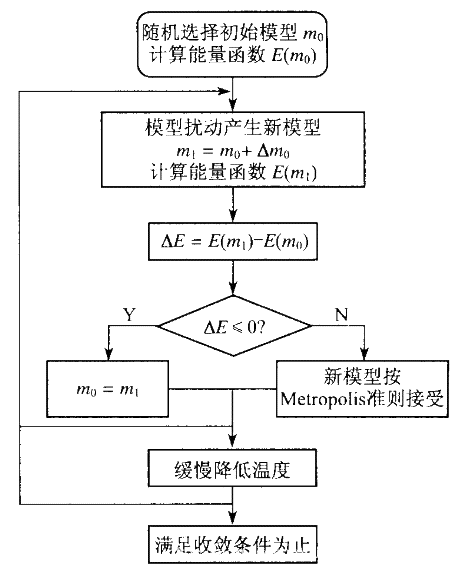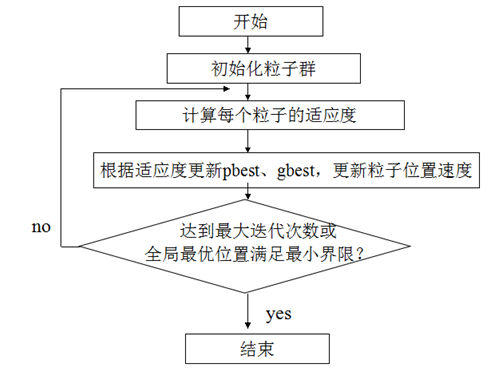# 智能算法-模拟退火-粒子群-鱼群算法

## 模拟退火算法

Metropolis准则
Metropolis法则是SA接受新解(扰动邻解)的概率。

$P(x=>x')=\begin{cases} 1 & f(x')<f(x) \\ e^{-\dfrac{f(x')-f(x)}{T}} & f(x')>=f(x) \end{cases}$

$T(t)=\dfrac{T_0}{log(1+t)}$

$T(t)=\dfrac{T_0}{1+t}$

$T(t+\Delta{t})=\alpha{T(t)}$

### 模拟退火算法流程

1. 初始化,温度T,任取一个初始解S1
2. 对当前温度T和k=1,2,…,L,重复步骤(3)~(6)
3. 对于当前的Si通过某种函数随机产生一个新解Si+1
4. 计算Si+1的花费增量df=f(Si+1)-f(Si),f为代价函数
5. 如果df<0,则用Si+1代替Si；否则按照exp(-df/T)的概率来决定是否用Si+1代替Si,（Metropolis准则）
6. 如果满足终止条件则退出,否则温度T衰减,返回步骤2## 粒子群算法

$V_{id}=\omega{V_{id}}+C_{1}random(0,1)(P_{id}-X_{id})+C_{2}random(0,1)(P_{id}-X_{id}) \\ X_{id}=X_{id}+V_{id}$

1. 初始化n个粒子的种群,初始化每个粒子的速度,位置
2. 设定最大速度Vmax
3. 计算每个个体的适应度,求得当前最优解
4. 根据最优解的位置,对每个粒子进行演化计算,算出每个粒子的速度(带方向)
5. 更具每个粒子的速度更新粒子的位置
6. 如果终止条件满足,则退出,否则返回3## 鱼群算法

1. 初始化设置,包括种群规模N、每条人工鱼的初始位置、人工鱼的视野Visual、步长step、拥挤度因子δ、重复次数T；
2. 计算初始鱼群各个体的适应值,取最优人工鱼状态及其值赋予Best；
3. 对每个个体进行评价Food Consistence,对其要执行的行为进行选择,包括觅食Pray、聚群Swarm、追尾Follow和随机行为；
4. 执行人工鱼的行为,更新自己,生成新鱼群；
5. 评价所有个体。若某个体优于Best ,则将Best更新为该个体；
6. 当满足终止条件时结束,否则转3

### 鱼群的个体行为

• 觅食行为：一般情况下鱼在水中随机地自由游动,当发现食物时,则会向食物逐渐增多的方向快速游去

• 聚群行为: 鱼在游动过程中为了保证自身的生存和躲避危害会自然地聚集成群,鱼聚群时所遵守的规则有三条:
– 分隔规则：尽量避免与临近伙伴过于拥挤;
– 对准规则：尽量与临近伙伴的平均方向一致;
– 内聚规则：尽量朝临近伙伴的中心移动。

• 追尾行为：当鱼群中的一条或几条鱼发现食物时,其临近的伙伴会尾随其快速到达食物点

• 随机行为：单独的鱼在水中通常都是随机游动的,这是为了更大范围地寻找食物点或身边的伙伴